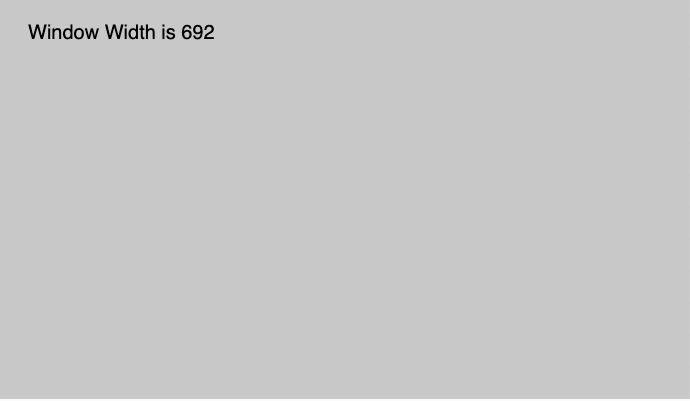GeeksforGeeks App
Open AppBrowser
Continue

## Related Articles

The windowWidth variable in p5.js is a system variable that is used to store the width of the inner window and it maps to window.innerWidth.
Syntax:

```windowWidth
```

Parameters: This function does not accept any parameter.

Below program illustrates the windowWidth variable in p5.js:
Example-1:

 `function` `setup() {``   ` `    ``createCanvas(1000, 400);``   ` `    ``// Set text size to 40px``    ``textSize(20);``}`` ` `function` `draw() {``    ``background(200);``   ` `    ``rect(mouseX, mouseY, 30, 30);``   ` `    ``//Use of windowWidth Variable``    ``text(``"Window Width is "` `+ windowWidth, 30, 40);``}`

Output:Example-2:

 `function` `setup() {`` ` `    ``// set height to window width ``    ``width = windowWidth;`` ` `    ``//create Canvas of size 380*80 ``    ``createCanvas(width, 100);``}`` ` `function` `draw() {``    ``background(220);``    ``textSize(16);``    ``textAlign(CENTER);``    ``fill(color(``'Green'``));`` ` `    ``//use of windowWidth variable``    ``text(``"windowWidth of Canvas is : "``         ``+ width, width / 2, height / 2);``}`

Output:My Personal Notes arrow_drop_up
Related Tutorials